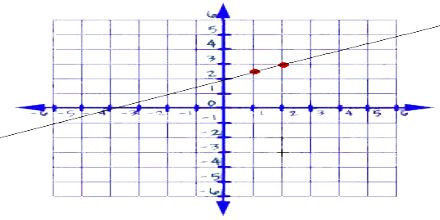Mathematic

# Linear EquationsThe aim of this lecture is to present on Linear Equations. Linear equations are functions which have two variables. They have an independent and dependent variable. Independent variables are variables that you put into the equations. Dependent variables are variables that you solve for. Just as common sense would dictate. If something is independent, then it is on its own. But if it is dependent, then it relies on someone or something else. A dependent variable relies on what is used in place of the independent variable